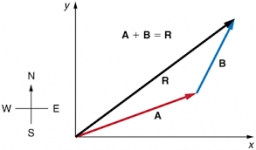# Displacement 55871

Assemble the two offsets d1 and d2 shown by OA and OB oriented lines. The coordinates of the points are O = (0m, 0m), A = (3m, 3m), B = (5m, 2m). Measure the magnitude of the resulting displacement d.

d =  9.434

### Step-by-step explanation:Did you find an error or inaccuracy? Feel free to write us. Thank you!

Tips for related online calculators
Our vector sum calculator can add two vectors given by their magnitudes and by included angle.
The Pythagorean theorem is the base for the right triangle calculator.

#### Grade of the word problem:

We encourage you to watch this tutorial video on this math problem: